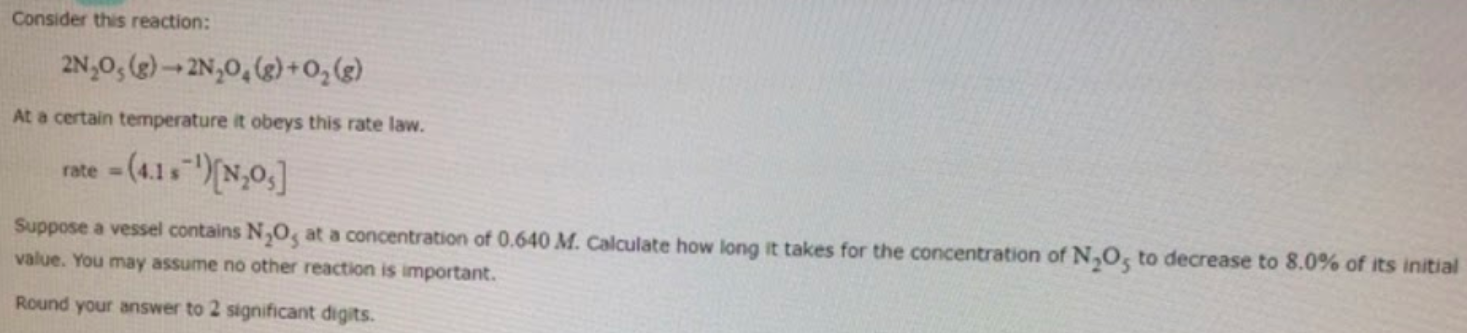# Consider this reaction: 2N2O5(g) → 2N2O4(g) + O2(g) At a certain temperature it obeys this rate law. rate = (4.1 s^-1)[N2O5] Suppose a vessel contains N2O5 at a concentration of 0.640 M. Calculate how long it takes for the concentration of N2O5 to decrease to 8.0% of its initial value. You may assume no other reaction is important. Round your answer to 2 significant digits.